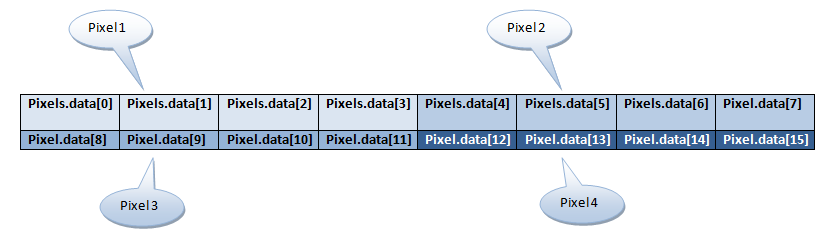# Iat455

## Workshops

### Working with Pixels

Bitmap: every bitmap image is represented as a... map of bits. 8 bits make a byte, and a byte is capable of storing values from 0-255 inclusive (or -127-128).

Take a look at this example of a 4 pixel image:In this image each row is 2 pixels long, and the 4th pixel is on the second row, second column, with 4 bytes per pixel. We can use this formula:

```        var bpc = 4; //bytes per pixel
var imgWidth = 2;
var pixelRow = 1;
var pixelCol = 1;
var pixel = pixelRow * bpc * imgWidth
+ pixelCol * bpc;
```

The pixels that represent an HTML5 Canvas element are stored in the data property of an ImageData object. The data property stores these pixels as a single dimension, interleaved array of byte values for each color channel: Red, Green, Blue, Alpha (RGBA).

So if you wanted the green value of the 4th pixel, you would use the formula above to figure out this would be the value at index 13 in the array: Pixels.data. Take a look at this example:

```        //get ImageData object using canvas 2d context
var imageData = context.getImageData(0, 0, context.canvas.width, context.canvas.height);
//get the green value of the 4th pixel
var pixel4greenChannel = imageData.data;
```

You can also change the values in this array directly but you must set the new ImageData object on the canvas 2d context in order to have a visible effect. Here's an example:

```      //make the 4th pixel black
for (var i = 0; i < 3; i++) {
imageData.data[12 + i] = 0;
}
imageData.data = 255; //full opacity
context.setImageData(imageData, 0, 0);
```

In your workshops, there is a lib called media.js which includes a straightforward way to work with canvas elements and their contexts like this:

```      //make a new Canvas object (not element)
var canvas = new Canvas();
//properties
canvas.context;
canvas.width;
canvas.height;
//methods
var imageData = canvas.getImageData();
canvas.setImageData(imageData);
```

There is another way to access pixels and it involves working with a single value as a 32 bit unsigned integer. This technique is faster than manipulating the data property of the ImageData object. Take a look:

```    var img = canvas.getImageData(),
data32 = new Uint32Array(img.data.buffer);
//looping all pixels
for (var i = 0; i < data32.length; i++) {
var pixel = data32[i];
//get the colors out of the pixel value
var r = (pixel) & 0xff,
g = (pixel >> 8) & 0xff,
b = (pixel >> 16) & 0xff;
a = (pixel >> 24) & 0xff;
//put the pixels back in (no change)
data32[i] = (a << 24)
| (b << 16)
| (g << 8)
| r;
}
```

The fancy operators here like |, &, and 0xff are just bitwise OR, AND, mask.